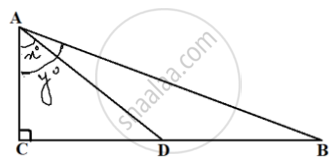# In the given figure, D is the mid-point of BC, then the value of coty∘cotx∘ is ______. - Mathematics

MCQ
Fill in the Blanks

In the given figure, D is the mid-point of BC, then the value of (coty^circ)/(cotx^circ) is ______.• 2

• 1/2

• 1/3

• 1/4

#### Solution

In the given figure, D is the mid-point of BC, then the value of (coty^circ)/(cotx^circ) is underline(1/2).

Explanation:-

(coty^circ)/(cotx^circ)=((AC)/(BC))/((AC)/(CD))=(CD)/(BC)=(CD)/(2CD)=1/2`

Concept: Areas of Similar Triangles
Is there an error in this question or solution?